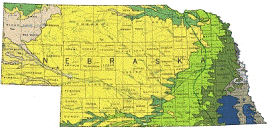## US Geological Survey## USGS Staff -- Published Research

#### Date of this Version

1992

Published in Hydraulic Engineering: Saving a Threatened Resource—In Search of Solutions: Proceedings of the Hydraulic Engineering sessions at Water Forum ’92. Baltimore, Maryland, August 2–6, 1992. Published by American Society of Civil Engineers.

#### Abstract

A numerical model is convergent if and only if a sequence of model solutions with increasingly refined solution domains approaches a fixed value. Furthermore, a numerical model is consistent only if this sequence converges to the solution of the continuous equations which govern the physical phenomenon being modeled. Given that a model is consistent, it is insufficient to apply it to a problem without testing for sensitivity to the size of the time and distance steps which form the discrete approximation of the solution domain. That is, convergence testing is a required component of any modeling study.

Two models were examined for this paper. The first model is a four-point implicit method applied to the unsteady one-dimensional open-channel flow equations. The second model is an unsteady one-dimensional or depth-averaged two-dimensional explicit diffusion-wave approximation of the shallow-water flow equations. Both models were applied to a one-dimensional channel problem. The two-dimensional model was applied to a simple two-dimensional flow field. Convergence testing is demonstrated in this paper by applying these models and examining the impact of increased spatial and temporal resolution on the results. It is demonstrated that both models are sensitive to changes in the spatial resolution and that erroneous solutions may result if this sensitivity is not understood during application of these models.

COinS